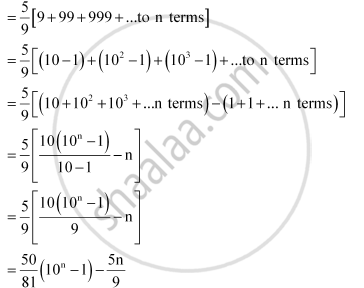CBSE (Arts) Class 11CBSE
Share
Notifications

View all notifications
Books Shortlist
Your shortlist is empty

# Find the Sum of the Following Series up To N Terms: 5 + 55 + 555 + … - CBSE (Arts) Class 11 - Mathematics

Login
Create free account

Forgot password?

#### Question

Find the sum of the following series up to n terms:

5 + 55 + 555 + …

#### Solution

5 + 55 + 555 + …

Let Sn = 5 + 55 + 555 + ….. to n termsIs there an error in this question or solution?

#### APPEARS IN

NCERT Solution for Mathematics Textbook for Class 11 (2018 to Current)
Chapter 9: Sequences and Series
Q: 21.1 | Page no. 200
Solution Find the Sum of the Following Series up To N Terms: 5 + 55 + 555 + … Concept: Concept of Series.
S